Participate in Codex, Test Your Skills & Win BIG

# C Interview Questions

C, one of the most popular computer languages today because of its structure, high-level abstraction, machine-independent feature, etc.

C language was developed to write the UNIX operating system, hence it is strongly associated with UNIX, which is one of the most popular network operating systems in use today and the heart of internet data superhighway.

In this article, you will understand the questions you could expect at a fresher, intermediate, and advanced level.

## C Interview Questions and Answers

### 1. What's the value of the expression 5["abxdef"]?

The answer is 'f'.

Explanation: The string mentioned "abxdef" is an array, and the  expression is equal to "abxdef". Why is the inside-out expression equivalent?  Because a[b] is equivalent to *(a + b) which is equivalent to *(b + a) which is equivalent to b[a].

### 2. What is a built-in function in C?

The most commonly used built-in functions in C are sacnf(), printf(), strcpy, strlwr, strcmp, strlen, strcat, and many more.

Built-function is also known as library functions are provided by the system to make the life of a developer easy by assisting them to do the certain commonly used predefined task. For example: if you need to print output or your program into the terminal we use printf() in C.

### 3. In C, What is the #line used for?

In C #line is used as a preprocessor to re-set the line number in the code, which takes a parameter as line number, herewith is an example for the same.

``````#include <stdio.h>								/*line 1*/
/*line 2*/
int main(){									/*line 3*/
/*line 4*/
printf("Nello world\n");					/*line 5*/
//print current line						/*line 6*/
printf("Line: %d\n",_LINE_);				/*line 7*/
//reset the line number by 36				/*line 8*/
#line 36	/*reseting*/
//print current line						/*line 36*/
printf("Line: %d\n",_LINE_);				/*line 37*/
printf("Bye bye!!!\n");						/*line 39*/
/*line 40*/
return 0;									/*line 41*/
}												/*line 42*/``````

### 4. How can a string be converted to a number?

The function takes the string as an input that needs to be converted to an integer.

``int atoi(const char *string)``

Return Value:

• On successful conversion, it returns the desired integer value
• If the string starts with alpha-numeric char or only contains alpha-num char, 0 is returned.
• In case string starts with numeric character but followed by alpha-num char, the string is converted to integer till the first occurrence of alphanumeric char.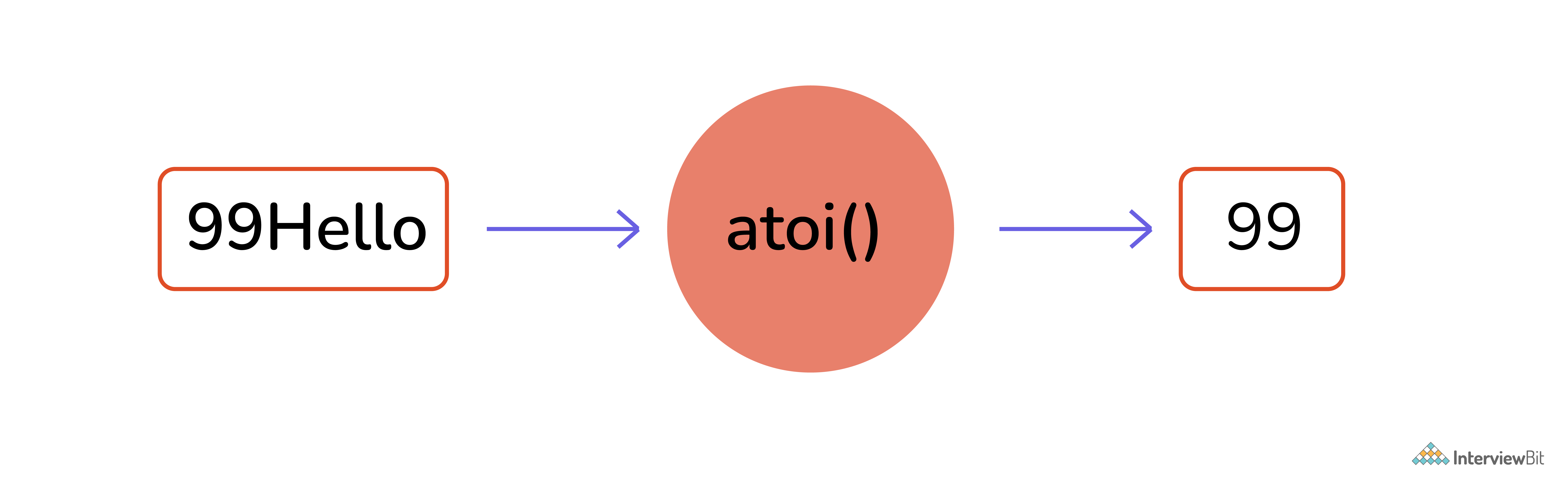Converting String to Number

### 5. How can a number be converted to a string?

The function takes a pointer to an array of char elements that need to be converted, and a format string needs to be written in a buffer as a string

``int sprintf(char *str, const char *format, ...)``
``````#include<stdio.h>
#include <math.h>
int main()
{
char str;

sprintf(str, "The value of PI = %f", M_PI);
puts(str);

return 0;
}``````

Below is the output after running the above code:

Output: Value of Pi = 3.141593

### 6. Why doesn’t C support function overloading?

After you compile the C source, the symbol names need to be intact in the object code. If we introduce function overloading in our source, we should also provide name mangling as a preventive measure to avoid function name clashes. Also, as C is not a strictly typed language many things(ex: data types) are convertible to each other in C, Therefore the complexity of overload resolution can introduce confusion in a language such as C.

When you compile a C source, symbol names will remain intact. If you introduce function overloading, you should provide a name mangling technique to prevent name clashes. Consequently, like C++, you'll have machine-generated symbol names in the compiled binary.

Additionally, C does not feature strict typing. Many things are implicitly convertible to each other in C. The complexity of overload resolution rules could introduce confusion in such kind of language

### 7. What is the difference between global int and static int declaration?

The difference between this is in scope. A truly global variable has a global scope and is visible everywhere in your program.

``````#include <stdio.h>

int my_global_var = 0;

int
main(void)

{
printf("%d\n", my_global_var);
return 0;
} ``````

global_temp is a global variable that is visible to everything in your program, although to make it visible in other modules, you'd need an ”extern int global_temp; ” in other source files if you have a multi-file project.

A static variable has a local scope but its variables are not allocated in the stack segment of the memory. It can have less than global scope, although - like global variables - it resides in the .bss segment of your compiled binary.

``````#include <stdio.h>

int
myfunc(int val)

{
static int my_static_var = 0;

my_static_var += val;
return my_static_var;
}

int
main(void)

{
int myval;

myval = myfunc(1);
printf("first call %d\n", myval);

myval = myfunc(10);

printf("second call %d\n", myval);

return 0;
}``````

### 8. Difference between const char* p and char const* p?

const char* p is a pointer to a const char.

char const* p is a pointer to a char const.

Since const char and char const are the same, it's the same.

### 9. Why n++ execute faster than n+1 ?

n++ being a unary operation that just needs one variable whereas n = n + 1 is a binary operation that adds overhead to take more time(Also binary operation: n += 1). However, in modern platforms, it depends on few things such as Processor Architecture, C Compiler, Usage in your code, Other factors such as hardware problems.

However in the modern compiler even if you write n = n + 1 it will get converted into n++ when it goes into the optimized binary, and it will be equivalently efficient.

### 10. What are the advantages of Macro over function?

Macro on a high-level copy-paste its definitions to places wherever it is called due to which it saves a lot of time, as no time is spent while passing the control to a new function as the control is always with the callee function. However, one downside is the size of the compiled binary is large but once compiled the program comparatively runs faster.

### 11. Specify different types of decision control statements?

All statements written in a program are executed from top to bottom one by one. Control statements are used to execute/transfer the control from one part of the program to another depending on the condition.

• If-else statement.
• normal if-else statement.
• Else-if statement
• nested if-else statement.
• Switch statement.

### 12. What is the difference between struct and union in C?

A struct is a group of complex data structure which is stored in a block of memory where each member on the block gets separate memory location which makes them accessible at once

Whereas in union all the member variables are stored at the same location on the memory as a result to which while assigning a value to a member variable will change the value of all other members.

``````/* struct & union definations*/
struct bar {
int a;	// we can use a & b both simultaneously
char b;
}	bar;

union foo {
int a;	// we can't use both a and b simultaneously
char b;
}	foo;

/* using struc and union variables*/

struct bar y;
y.a = 3;	// OK to use
y.b = 'c'; // OK to use

union foo x;
x.a = 3; // OK
x.b = 'c'; // NOl this affects the value of x.a!``````

### 13. What is call by reference in functions?

When we caller function makes a function call bypassing the addresses of actual parameters being passed, then this called call by reference. Incall by reference, the operation performed on formal parameters affects the value of actual parameters because all the operations performed on the value stored in the address of actual parameters.

### 14. What is pass by reference in functions?

In Pass by reference, the callee receives the address and makes a copy of the address of an argument into the formal parameter. Callee function uses the address to access the actual argument (to do some manipulation). If the callee function changes the value addressed at the passed address it will be visible to the caller function as well.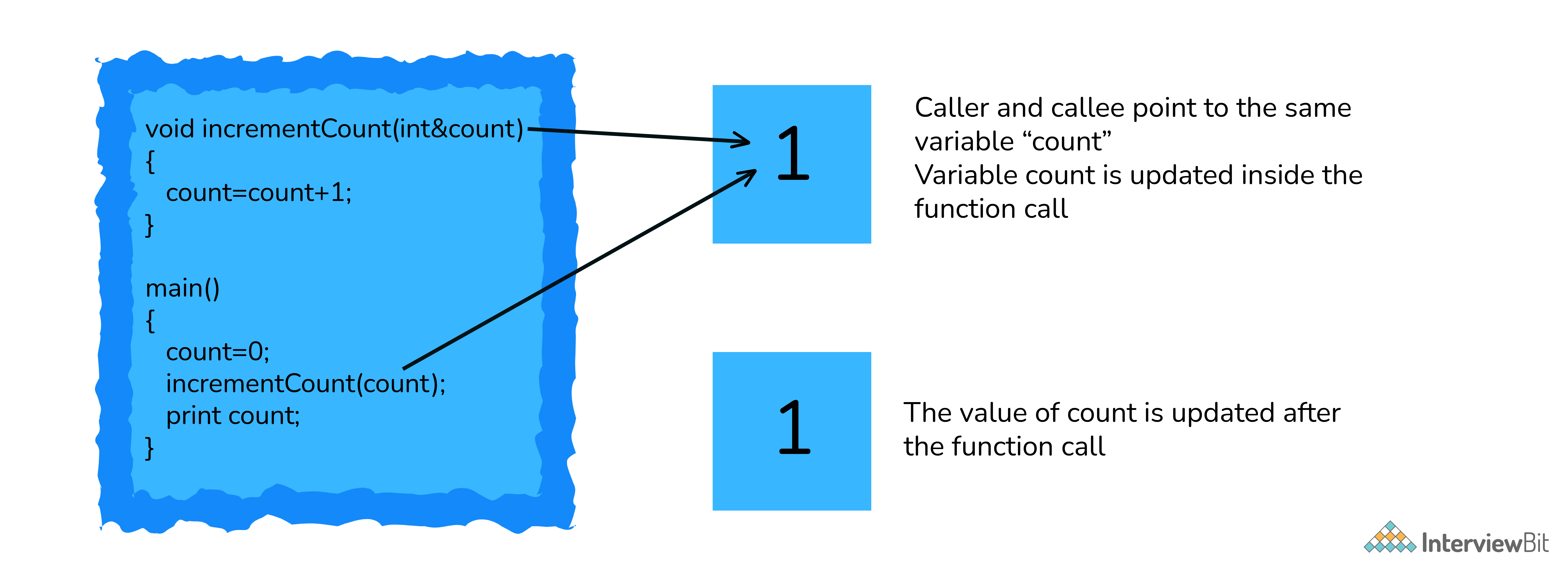Pass By Reference

### 15. What is a memory leak? How to avoid it?

When we assign a variable it takes space of our RAM (either heap or RAM)dependent on the size of data type, however, if a programmer uses a memory available on the heap and forgets to a delta it, at some point all the memory available on the ram will be occupied with no memory left this can lead to a memory leak.

``````int main()
{
char * ptr = malloc(sizeof(int));

/* Do some work */
/*Not freeing the allocated memory*/
return 0;
}``````

To avoid memory leaks, you can trace all your memory allocations and think forward, where you want to destroy (in a good sense) that memory and place delete there. Another way is to use C++ smart pointer in C linking it to GNU compilers.

### 16. What is Dynamic memory allocation in C? Name the dynamic allocation functions.

C is a language known for its low-level control over the memory allocation of variables in DMA there are two major standard library malloc() and free. The malloc() function takes a single input parameter which tells the size of the memory requested It returns a pointer to the allocated memory. If the allocation fails, it returns NULL. The prototype for the standard library function is like this:

void *malloc(size_t size);
The free() function takes the pointer returned by malloc() and de-allocates the memory. No indication of success or failure is returned. The function prototype is like this:

void free(void *pointer);
There are 4 library functions provided by C defined under <stdlib.h> header file to facilitate dynamic memory allocation in C programming. They are:

• malloc()
• calloc()
• free()
• realloc()

### 17. What is typedef?

typedef is a C keyword, used to define alias/synonyms for an existing type in C language. In most cases, we use typedef's to simplify the existing type declaration syntax. Or to provide specific descriptive names to a type.

``typedef <existing-type> <new-type-identifiers>;``

typedef provides an alias name to the existing complex type definition. With typedef, you can simply create an alias for any type. Whether it is a simple integer to complex function pointer or structure declaration, typedef will shorten your code.

### 18. Why is it usually a bad idea to use gets()? Suggest a workaround.

The standard input library gets() reads user input till it encounters a new line character. However, it does not check on the size of the variable being provided by the user is under the maximum size of the data type due to which makes the system vulnerable to buffer overflow and the input being written into memory where it isn’t supposed to.

We, therefore, use fgets() to achieve the same with a restricted range of input

Bonus: It remained an official part of the language up to the 1999 ISO C standard, but it was officially removed by the 2011 standard. Most C implementations still support it, but at least GCC issues a warning for any code that uses it.

### 19. What is the difference between #include "..." and #include <...>?

In practice, the difference is in the location where the preprocessor searches for the included file.

For #include <filename> the C preprocessor looks for the filename in the predefined list of system directories first and then to the directories told by the user(we can use -I option to add directories to the mentioned predefined list).

For #include "filename" the preprocessor searches first in the same directory as the file containing the directive, and then follows the search path used for the #include <filename> form. This method is normally used to include programmer-defined header files.

### 20. What are dangling pointers? How are dangling pointers different from memory leaks?

The dangling pointer points to a memory that has already been freed. The storage is no longer allocated. Trying to access it might cause a Segmentation fault. A common way to end up with a dangling pointer:

``````#include<stdio.h>
#include<string.h>

char *func()
{
char str;
strcpy(str,"Hello!");
return(str);
}``````

You are returning an address that was a local variable, which would have gone out of scope by the time control was returned to the calling function. (Undefined behavior)

``````*c = malloc(5izeof(int));
free(c);
*c = 3; //writing to freed location!``````

In the figure shown above writing to a memory that has been freed is an example of the dangling pointer, which makes the program crash.

A memory leak is something where the memory allocated is not freed which causes the program to use an undefined amount of memory from the ram making it unavailable for every other running program(or daemon) which causes the programs to crash. There are various tools like O profile testing which is useful to detect memory leaks on your programs.

``````void function(){
char *leak = malloc (10);	//leak assigned but not freed
}``````

### 21. What is the difference between ‘g’ and “g” in C?

In C double-quotes variables are identified as a string whereas single-quoted variables are identified as the character. Another major difference being the string (double-quoted) variables end with a null terminator that makes it a 2 character array.

## C Interview Questions For Experienced

### 22. Can you tell me how to check whether a linked list is circular?

Single Linked List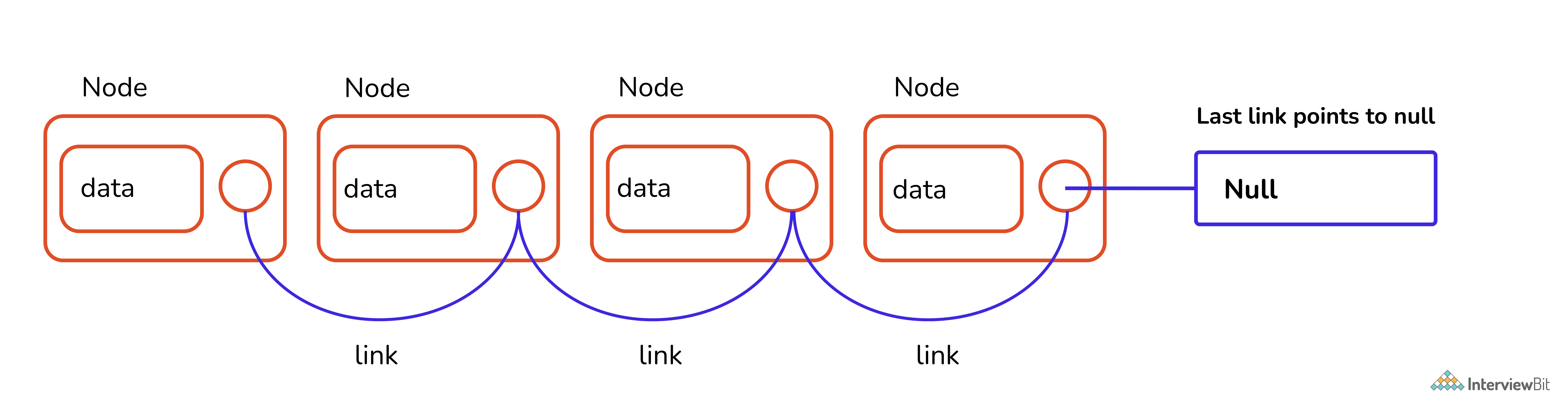Single Linked List

Circular Linked List

Circular linked list is a variation of a linked list where the last node is pointing to the first node's information part. Therefore the last node does not point to null.

### Algorithm to find whether the given linked list is circular

A very simple way to determine whether the linked list is circular or not

• Traverse the linked list
• Check if the node is pointing to the head.
• If yes then it is circular.

Let's look at the snippet where we code this algorithm.

``````Create a structure for a linked list
Declare
-Variable to store data of the node.
-Pointer variable of struct type to store the address of next node.

function of datatype tool isCircular(firstgode){

-Store the value of first node in temp variable and make it traverse all nodes.
-temp-firstgode
-tempenext node pointed by temp(temp->next)
-run until temp is at null or firstNode

if (temp at null)
not circular and returns false
if (temp points first node)
return true as its circular.
}

function of datatype node newNode(data){

-To insert new nodes and link each one of them to the previous node by storing the address of the new node to the previous one.
-Then make them point to NULL.
}

In int main function

-First insert nodes for circular linked list and check its nature by calling isCircular function.
-Since it is true through if statement it prints "yes..
-Second insert a normal linked list and check its nature by calling isCircular function. As its not circular it prints "no",``````

### 23. What is the use of a semicolon (;) at the end of every program statement?

It is majorly related to how the compiler reads( or parses) the entire code and breaks it into a set of instructions(or statements), to which semicolon in C acts as a boundary between two sets of instructions.

### 24. Differentiate Source Codes from Object Codes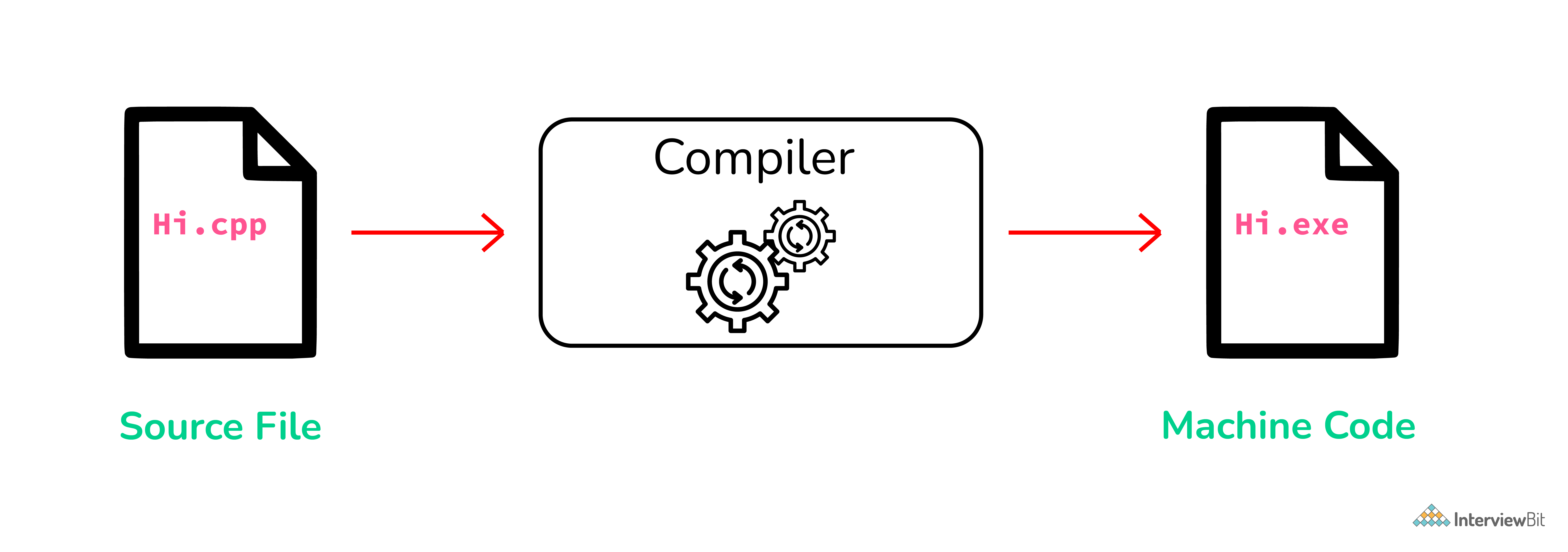Source Code and Object Code Difference

The difference between the Source Code and Object Code is that Source Code is a collection of computer instructions written using a human-readable programming language while Object Code is a sequence of statements in machine language, and is the output after the compiler or an assembler converts the Source Code.

The last point about Object Code is the way the changes are reflected. When the Source Code is modified, each time the Source Code needs to be compiled to reflect the changes in the Object Code.

### 25. What are header files and what are its uses in C programming?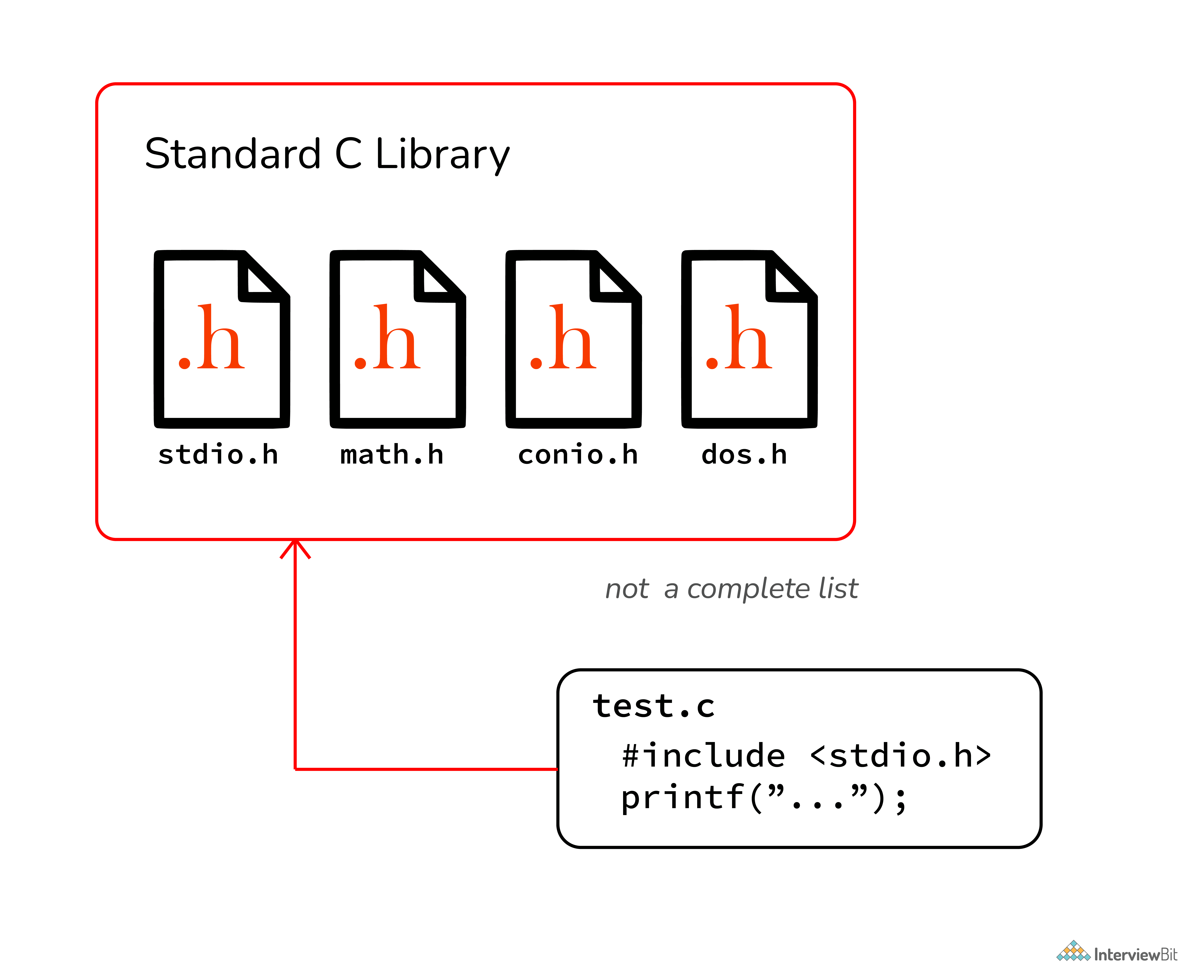Header Files in C

In C header files must have the extension as .h, which contains function definitions, data type definitions, macro, etc. The header is useful to import the above definitions to the source code using the #include directive. For example, if your source code needs to take input from the user do some manipulation and print the output on the terminal, it should have stdio.h file included as #include <stdio.h>, with which we can take input using scanf() do some manipulation and print using printf().

### 26. When is the "void" keyword used in a function

The keyword “void” is a data type that literally represents no data at all. The most obvious use of this is a function that returns nothing:

``````void PrintHello()
{
printf("Hello\n");
return;		// the function does "return", but no value is returned
} ``````

Here we’ve declared a function, and all functions have a return type. In this case, we’ve said the return type is “void”, and that means, “no data at all” is returned.
The other use for the void keyword is a void pointer. A void pointer points to the memory location where the data type is undefined at the time of variable definition. Even you can define a function of return type void* or void pointer meaning “at compile time we don’t know what it will return” Let’s see an example of that.

``````void MyMemCopy(void* dst, const void* src, int numBytes)
{
char* dst_c = reinterpret_cast<char*>(dst);
const char* src_c = reinterpret_cast<const char*>(src);
for (int i = 0; i < numBytes; ++i)
dst_c[i] = src_c[i];
} ``````

### 27. What is dynamic data structure?

A dynamic data structure (DDS) refers to an organization or collection of data in memory that has the flexibility to grow or shrink in size, enabling a programmer to control exactly how much memory is utilized. Dynamic data structures change in size by having unused memory allocated or de-allocated from the heap as needed.

Dynamic data structures play a key role in programming languages like C, C++, and Java because they provide the programmer with the flexibility to adjust the memory consumption of software programs.

### 28. Add Two Numbers Without Using the Addition Operator

For the sum of two numbers, we use the addition (+) operator. In these tricky C programs, we will write a C program to add two numbers without using the addition operator.

``````#include<stdio.h>
#include<stdlib.h>
int main()
{
int x, y;
printf("Enter two number: ");
scanf("%d %d",&x,&y);

// method 1
printf("%d\n", x-(-y));

// method 2
printf("%d\n", -(-x-y));

// method 3
printf("%d\n", abs(-x-y));

// method 4
printf("%d", x-(~y)-1);

return 0;
}``````

### 29. Subtract Two Number Without Using Subtraction Operator

``````#include<stdio.h>
#include<stdlib.h>
int main()
{
int x, y;
printf("Enter two number: ");
scanf("%d %d",&x,&y);
printf("%d", x+(~y)+1);
return 0;
}``````

The bitwise complement operator is used in this program. The bitwise complement of number ~y=-(y+1). So, expression will become x+(-(y+1))+1=x-y-1+1=x-y

### 30. Multiply an Integer Number by 2 Without Using Multiplication Operator

``````#include<stdio.h>
int main()
{
int x;
printf("Enter a number: ");
scanf("%d",&x);
printf("%d", x<<1);
return 0;
}``````

The left shift operator shifts all bits towards the left by a certain number of specified bits. The expression x<<1 always returns x*2. Note that the shift operator doesn’t work on floating-point values.

For multiple of x by 4, use x<<2. Similarly x<<3 multiply x by 8. For multiple of the number x by 2^n, use x<<n.

### 31. Check whether the number is EVEN or ODD, without using any arithmetic or relational operators

``````#include<stdio.h>
int main()
{
int x;
printf("Enter a number: ");
scanf("%d", &x);
(x&1)?printf("Odd"):printf("Even");
return 0;
}``````

The bitwise and(&) operator can be used to quickly check the number is odd or even.

### 32. Reverse the Linked List. Input: 1->2->3->4->5->NULL Output: 5->4->3->2->1->NULL

Assume that we have linked list 1 → 2 → 3 → Ø, we would like to change it to Ø ← 1 ← 2 ← 3.While you travel the linked list, change the current node's next pointer to point to its previous element. reference to the previous nodes should be stored into a temp variable as shown so that we don’t lose track of the swapped node. You also need another pointer to store the next node before changing the reference. Also when we are done return the new head of the reversed list.

``````/* Function to reverse the linked list */
static void reverse(struct Node** head_ref)
{
struct Node* prev   = NULL;
struct Node* current = *head_ref;
struct Node* next;
while (current != NULL)
{
// store next
next  = current->next;

// reverse curr node pointer
current->next = prev;

// move pointer one position ahead
prev = current;
current = next;
}
*head_ref = prev;
}``````

### 33. Check for Balanced Parentheses using Stack

Given a string s containing just the characters '(', ')', '{', '}', '[' and ']', determine if the input string is valid.

An input string is valid if:

• Open brackets must be closed by the same type of brackets.
• Open brackets must be closed in the correct order.

Example 1:
Input: s = "()"
Output: true

Example 2:
Input: s = "()[]{}"
Output: true

Example 3:
Input: s = "(]"
Output: falseBelow is the source code for C Program to Check for Balanced Parentheses using Stack which is successfully compiled and run on Windows System to produce desired output as shown below :

``````int check(char exp[] )
{
int i;
char temp;
for(i=0;i<strlen(exp);i++)
{
if(exp[i]=='(' || exp[i]=='{' || exp[i]=='[')
push(exp[i]);
if(exp[i]==')' || exp[i]=='}' || exp[i]==']')
if(top==-1)    /*stack empty*/
{
printf("Right parentheses are more than left parentheses\n");
return 0;
}
else
{
temp=pop();
if(!match(temp, exp[i]))
{
printf("Mismatched parentheses are : ");
printf("%c and %c\n",temp,exp[i]);
return 0;
}
}
}
if(top==-1) /*stack empty*/
{
printf("Balanced Parentheses\n");
return 1;
}
else
{
printf("Left parentheses more than right parentheses\n");
return 0;
}
}``````

### 34. Program to find n’th Fibonacci number

Fibonacci sequence is characterized by the fact that every number after the first two is the sum of the two preceding ones. For example, consider below sequence

0, 1, 1, 2, 3, 5, 8, 13, 21, 34, . .. and so on

Where in F{n} = F{n-1} + F{n-2} with base values F(0) = 0 and <code>F(1) = 1

Below is naive implementation for finding the nth member of the Fibonacci sequence

``````// Function to find the nth Fibonacci number
int fib(int n)
{
if (n <= 1) {
return n;
}

return fib(n - 1) + fib(n - 2);
}

int main()
{
int n = 8;

printf("nth Fibonacci number is %d", fib(8));

return 0;
}``````

### 35. Write a program to find the node at which the intersection of two singly linked lists begins.

Let's take an example of the following two linked lists which intersect at node c1.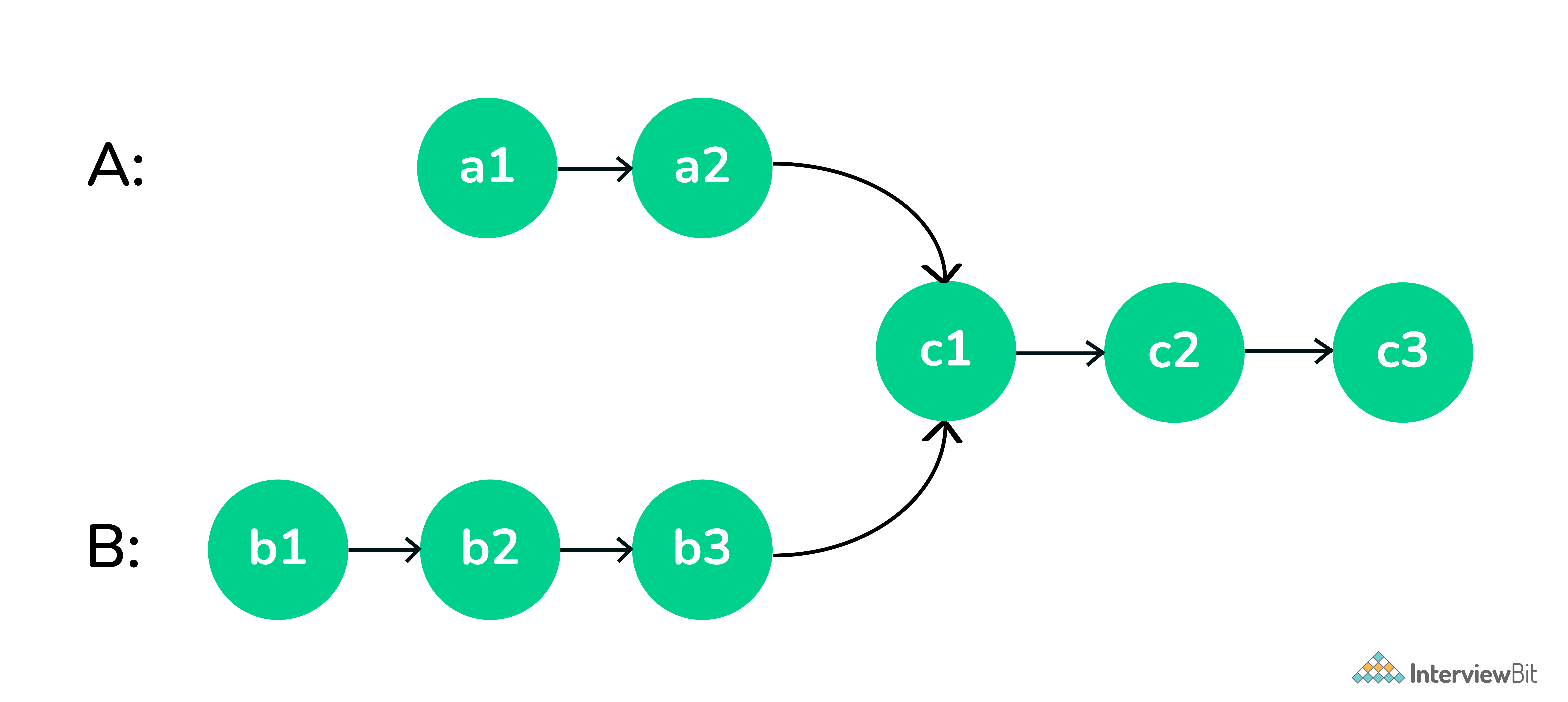Intersection of Two Linked List

Solution -

• Get count of the nodes in the first list, let count be c1.
• Get count of the nodes in the second list, let count be c2.
• Get the difference of counts d = abs(c1 – c2)
• Now traverse the bigger list from the first node till d nodes so that from here onwards both the lists have an equal no of nodes
• Then we can traverse both the lists in parallel till we come across a common node. (Note that getting a common node is done by comparing the address of the nodes)
``````// Function to get the intersection point
// of the given linked lists
int getIntersectionNode(Node* head1, Node* head2)
{
Node *curr1 = head1, *curr2 = head2;

// While both the pointers are not equal
while (curr1 != curr2) {

// If the first pointer is null then
// set it to point to the head of
// the second linked list
if (curr1 == NULL) {
curr1 = head2;
}

// Else point it to the next node
else {
curr1 = curr1->next;
}

// If the second pointer is null then
// set it to point to the head of
// the first linked list
if (curr2 == NULL) {
curr2 = head1;
}

// Else point it to the next node
else {
curr2 = curr2->next;
}
}

// Return the intersection node
return curr1->data;
}``````

### 36. Merge Two sorted Linked List

Merge two sorted linked lists and return them as a sorted list. The list should be made by splicing together the nodes of the first two lists.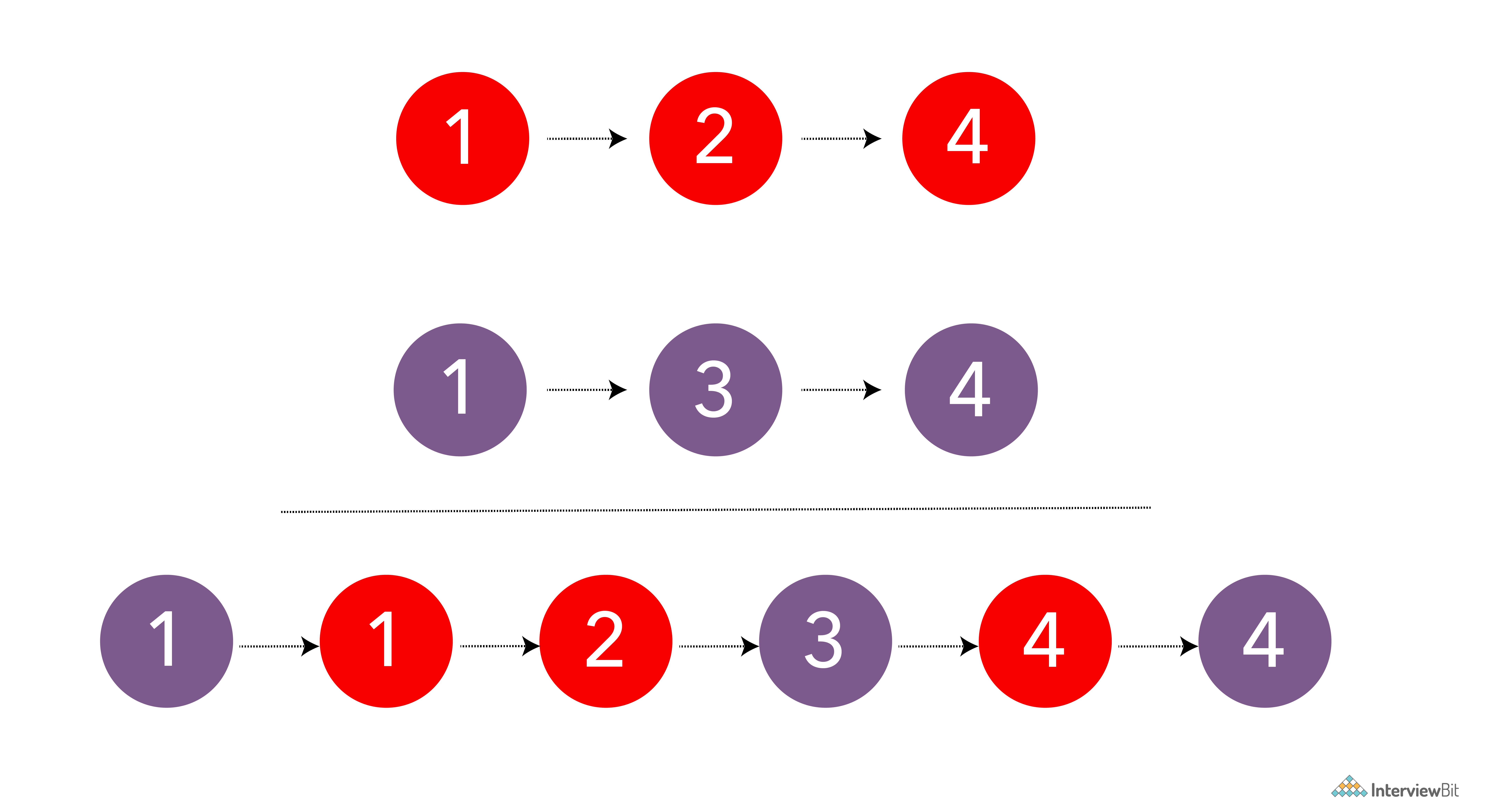Merging Two Sorted Linked List
``````NodePtr merge_sorted(NodePtr head1, NodePtr head2) {

// if both lists are empty then merged list is also empty
// if one of the lists is empty then other is the merged list
if (head1 == nullptr) {
return head2;
} else if (head2 == nullptr) {
return head1;
}

NodePtr mergedHead = nullptr;
if (head1->data <= head2->data) {
mergedHead = head1;
head1 = head1->next;
} else {
mergedHead = head2;
head2 = head2->next;
}

NodePtr mergedTail = mergedHead;

while (head1 != nullptr && head2 != nullptr) {
NodePtr temp = nullptr;
if (head1->data <= head2->data) {
temp = head1;
head1 = head1->next;
} else {
temp = head2;
head2 = head2->next;
}

mergedTail->next = temp;
mergedTail = temp;
}

if (head1 != nullptr) {
mergedTail->next = head1;
} else if (head2 != nullptr) {
mergedTail->next = head2;
}

return mergedHead;
}``````

Runtime Complexity Linear, O(m + n) where m and n are lengths of both linked lists.
Memory Complexity Constant, O(1)

Maintain a head and a tail pointer on the merged linked list. Then choose the head of the merged linked list by comparing the first node of both linked lists. For all subsequent nodes in both lists, you choose the smaller current node and link it to the tail of the merged list, and moving the current pointer of that list one step forward.

You keep doing this while there are some remaining elements in both the lists. If there are still some elements in only one of the lists, you link this remaining list to the tail of the merged list.

Initially, the merged linked list is NULL. Compare the value of the first two nodes and make the node with the smaller value the head node of the merged linked list. In this example, it is 4 from head1.

Since it’s the first and only node in the merged list, it will also be the tail. Then move head1 one step forward.

## C Coding Questions

1.

C variable cannot start with which of the following option

2.

During function call, Default Parameter Passing Mechanism is called as

3.

The statement printf(“%d”, 10 ? 0 ? 5 : 1 : 12); will print?

4.

In the switch statement, each case instance value must be _______?

5.

Which is the correct syntax to declare constants in C?

6.

Bitwise operators can operate upon?

7.

The number of binary trees that can be formed using 5 nodes are

8.

What is the correct syntax to access the value of struct variable book{ price, page }?

9.

Which of the following data structures is linear type?

10.

A binary tree with 27 nodes has _______ null branches.